Next: Exercises Up: lab_template Previous: lab_template

Subsections

# Area Approximations

## Purpose

The purpose of this lab is to acquaint you with some rectangular approximations to areas under curves.

## Background

Integration, the second major theme of calculus, deals with areas, volumes, masses, and averages such as centers of mass and gyration. In lecture you have learned that the area under a curve between two points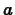and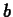can be found as a limit of a sum of areas of rectangles which are in some sense under'' the curve of interest. As these sums, and their limits, are often tedious to calculate, there is clear motivation for the analytical techniques which will be introduced shortly in class. However, not all area finding'' problems can be solved using analytical techniques, and the Riemann sum definition of area under a curve gives rise to several numerical methods which can often approximate the area of interest with great accuracy.

Suppose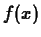is a non-negative, continuous function defined on some interval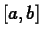. Then by the area under the curve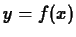between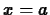andwe mean the area of the region bounded above by the graph of, below by the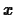axis, on the left by the vertical line, and on the right by the vertical line.

All of the numerical methods in this lab depend on subdividing the intervalinto subintervals of uniform length. For example, dividing the interval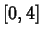into four uniform pieces produces the subintervals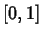,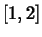,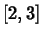, and.

### Rectangular approximations

In these simple approximation schemes, the area above each subinterval is approximated by the area of a rectangle, with the height of the rectangle being chosen according to some rule. In particular, we will consider the following rules:
left endpoint rule
The height of the rectangle is the value of the functionat the left-hand endpoint of the subinterval.

right endpoint rule
The height of the rectangle is the value of the functionat the right-hand endpoint of the subinterval.

midpoint rule
The height of the rectangle is the value of the functionat the midpoint of the subinterval.

The Maple student package has commands for visualizing these three rectangular area approximations. To use them, you first must load the package via the with command. Then try the three commands given below. Make sure you understand the differences between the three different rectangular approximations. Take a moment to see that the different rules choose rectangles which in each case will either underestimate or overestimate the area.

  >
with(student):


  >
rightbox(x^2,x=0..4);


  >
leftbox(x^2,x=0..4);


  >
middlebox(x^2,x=0..4);


There are also Maple commands leftsum, rightsum, and middlesum to sum the areas of the rectangles, see the examples below. Note the use of evalf to obtain numerical answers.

  >
rightsum(x^2,x=0..4);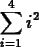>
evalf(rightsum(x^2,x=0..4));>
middlesum(x^2,x=0..4);>
evalf(middlesum(x^2,x=0..4));It should be clear from the graphs that adding up the areas of the rectangles only approximates the area under the curve. However, by increasing the number of subintervals the accuracy of the approximation can be increased. All of the Maple commands described so far in this lab permit a third argument to specify the number of subintervals. The default is 4 subintervals. See the example below for appoximations to the area under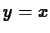from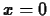to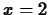using the rightsum command with 4, 10, 20 and 100 subintervals. (As this region describes a right triangle with height 2 and base 2, this area can be easily calculated to be exactly 2.) Try it yourself with the leftsum and middlesum commands.

  >
evalf(rightsum(x,x=0..2));>
evalf(rightsum(x,x=0..2,10));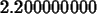>
evalf(rightsum(x,x=0..2,20));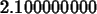>
evalf(rightsum(x,x=0..2,100));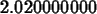Since, in this trivial example, we knew that the area is exactly 2, it appears that, as the number of subintervals increases, the rectangular approximation becomes more accurate. What would happen with a not so trivial region? The next section describes a way of assessing the accuracy of the midpoint rule.

### Error bound for the midpoint rule

The midpoint rule with n subintervals (designated as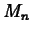) usually gives better accuracy than either the left endpoint rule (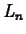) or the right endpoint rule (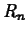). This means that, for a given n,is generally closer to A than eitheror. In numerical analysis texts it is shown that the error,, in usingto approximate the area underonsatisfies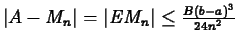where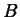is the absolute maximum ofon, assuming that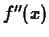is continuous on that interval. In practice,is often approximated by a number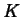that is an upper bound for, that is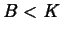. For instance, if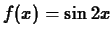on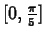,might be taken as 4. Do you see why? For more complicated functions, Maple can be used to get a value forthat is close to or actually equal to. Note that the error bound formula gives a worst case estimate, the accuracy achieved for a given number of subintervals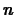may be much better than the guarantee given by the formula.Next: Exercises Up: lab_template Previous: lab_template
Dina Solitro
2001-01-12